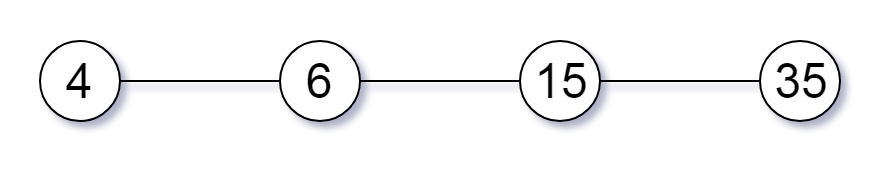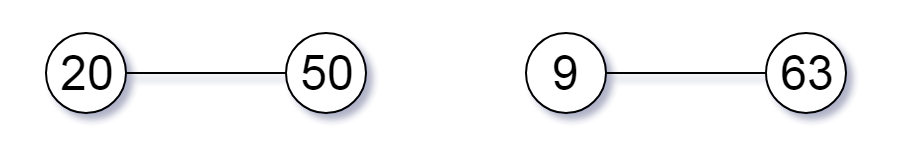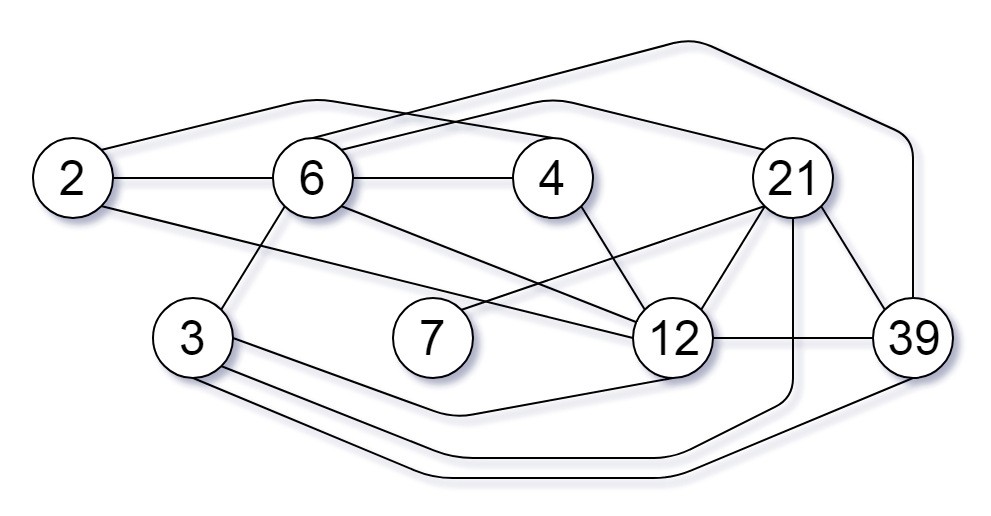# GeetCode Hub

Given a non-empty array of unique positive integers `nums`, consider the following graph:

• There are `nums.length` nodes, labelled `nums` to `nums[nums.length - 1];`
• There is an edge between `nums[i]` and `nums[j]` if and only if `nums[i]` and `nums[j]` share a common factor greater than 1.

Return the size of the largest connected component in the graph.

Example 1:

```Input: nums = [4,6,15,35]
Output: 4```

Example 2:

```Input: nums = [20,50,9,63]
Output: 2```

Example 3:

```Input: nums = [2,3,6,7,4,12,21,39]
Output: 8```

Note:

1. `1 <= nums.length <= 20000`
2. `1 <= nums[i] <= 100000`

class Solution { public int largestComponentSize(int[] nums) { } }